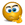# Nonlinear regression using excel's data analysis tool

## Excel Help for Nonlinear Regression Using Excel's Data Analysis Tool in Excel Multiple Regression Analysis and Forecasting Template

### Nonlinear Regression Using Excel's Data Analysis Tool

Rate this:I’m trying to understand how to make use of the equation suggested by excel when fitting a trendline through the following set of data

 x y 2004 17% 2005 19% 2006 22% 2007 24% 2008 25% 2009 26%

and selecting the polynomial curve as the best fit. The regression returns the following coefficients:

Y=-0.0022x^2 +8.8713x-8919.6

So to evaluate Y when x=2010 I would calculate:

Y=-0.0022*(2010)^2 +8.8713*2010-8919.6

Which results in Y being equal to 23.493  which is non sensible and doesn’t match the Y value the graph would imply for the year 2010 (something around 27%).

Can somebody please tell me what I’m doing wrong?
Posted by on

 Replies - Displaying 1 to 3 of 3 Order Replies By: Most Recent | Chronological | Highest RatedRate this: (3/5 from 2 votes) Hithe software take x as 1,2,3,4,5,6 not as you expecet as 2004,2005......2009then if you aplay x=7    (which should be 2010)the result will be close to what you expect All the best - m.saad Posted by msaad on 14 Nov 2010Rate this: (3/5 from 1 vote) That makes perfect sense actually. Thanks so much! Posted by Catrina on 15 Nov 2010Rate this: (4/5 from 2 votes) Setting up a nonlinear regressionAfter opening XLSTAT, select the XLSTAT / Modeling data / Nonlinear regression command. The nonlinear regression dialog box pops up. Select the data on the Excel sheet. The Dependent variable (or variable to model, or response variable) is in our case the "Speed".Regards,Rachel Gomez rachel Posted by RachelGomez161999 on 09 Dec 2022
 Displaying page 1 of 1

 Find relevant Excel templates and add-ins for Nonlinear regression using excel's data analysis tool in the Excel Business Solutions Directory